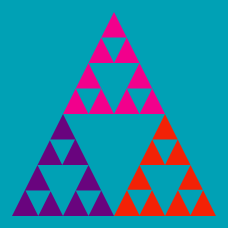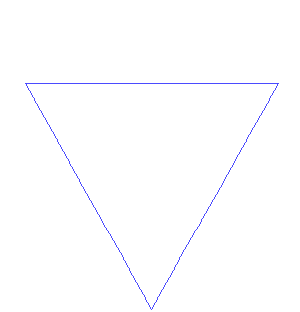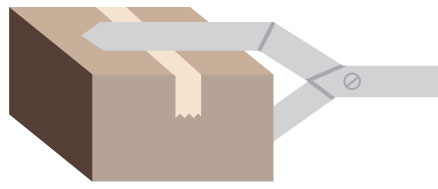Probability

# Introduction to Recursion: Level 3 ChallengesWhat is the area enclosed by a Koch snowflake starting from an equilateral triangle with side length 1?

A. 1
B. $\frac{1}{2}$
C. $\frac{2\sqrt{3}}{5}$
D. $2 \frac{\sqrt{3}}{4}$
E. Area is infiniteAt a warehouse, robotic arms are responsible for retrieving boxes when an order is received from a customer. In one area of the warehouse, there is a stack of 6 boxes. Each box is a cube, and all the boxes have different sizes. The boxes are stacked from largest to smallest, with the largest box on the bottom.

When doing an inventory check, the warehouse foreman realizes that this stack of boxes is in the wrong spot. There are two empty spots to the right of this stack, and the boxes are supposed to be in the rightmost spot. The foreman programs the robotic arm to move the boxes from where there are now into the new stack. The robotic arm is only able to pick up one box at a time, and for safety reasons, it is never allowed to place any box on top of a smaller box, since it might fall off.

What is the smallest number of moves that the robotic arm must make in order to move the stack of boxes from its present location to where it is supposed to be?

\begin{aligned} A&=&2000\\ \\ B&=&A-999\\ \\ C&=&A+B-998\\ \\ D&=&A+B+C-997\\ & \vdots & \\ Z&=&A+B+C+...+Y-975 \end{aligned}

How much is $\frac { Z+1 }{ { 2 }^{ 25 } } ?$

Define a sequence such that $A_1 = 2$ and $A_{n+1}$ is the sum of the squares of the digits of $A_n.$ Determine the value of $A_{1999} + A_{2000}$.

Let there be a sequence $a_1, a_2, a_3 \dots \dots a_{2014}$. Let $a_1$ be $2014$. If the next number in the series is the sum of the cubes of the digits of the previous number, what is the $2014^{\text{th}}$ number in the sequence?

×